# Quadratic Functions Solving by Graphing Quadratic Function Standard

• Slides: 15Quadratic Functions Solving by GraphingQuadratic Function Standard Form: f(x) = ax 2 + bx + c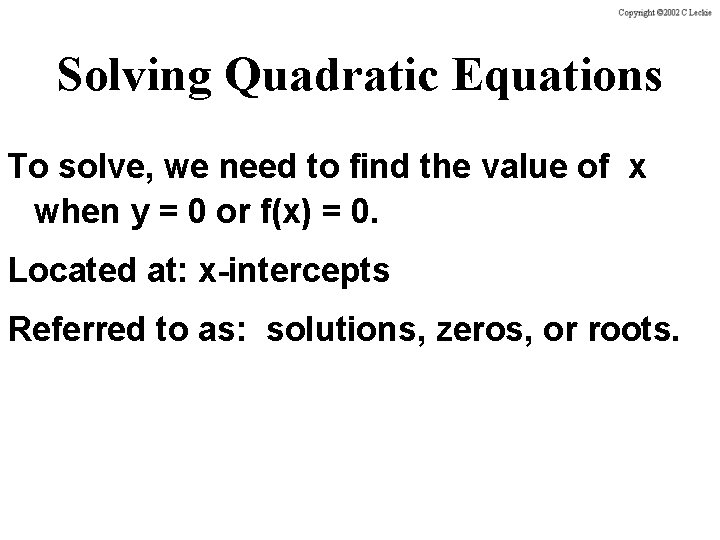Solving Quadratic Equations To solve, we need to find the value of x when y = 0 or f(x) = 0. Located at: x-intercepts Referred to as: solutions, zeros, or roots.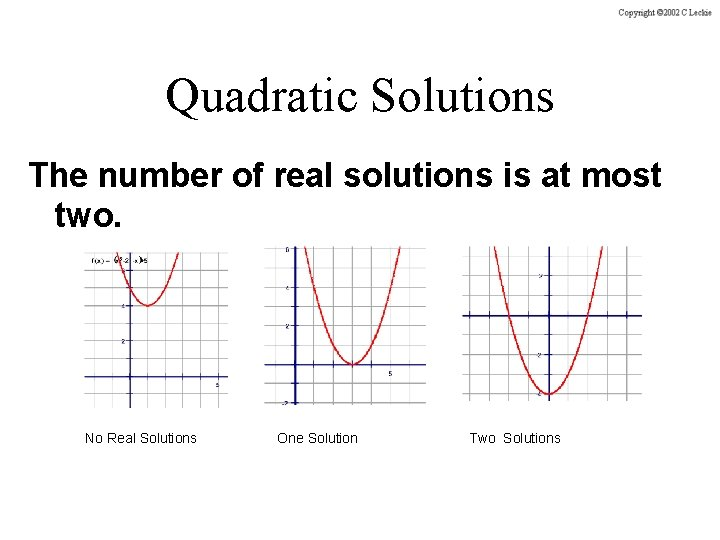Quadratic Solutions The number of real solutions is at most two. No Real Solutions One Solution Two SolutionsIdentifying Solutions 2 Example f(x) = x - 4 Solutions are x = -2 and x = 2.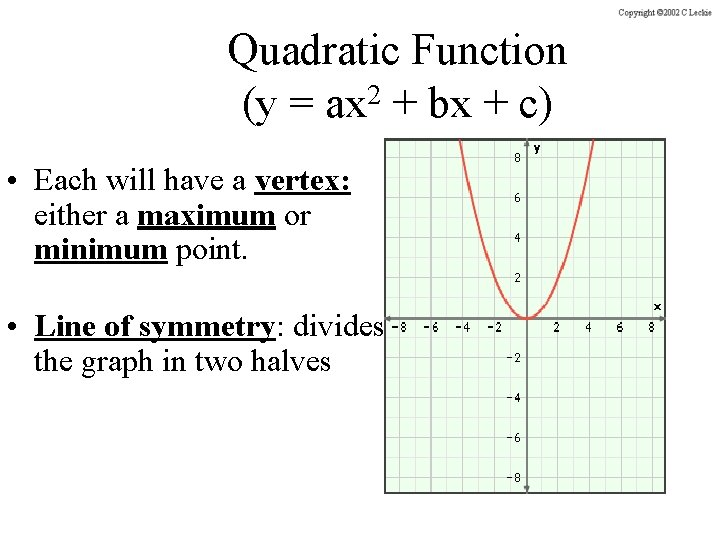Quadratic Function 2 (y = ax + bx + c) • Each will have a vertex: either a maximum or minimum point. • Line of symmetry: divides the graph in two halves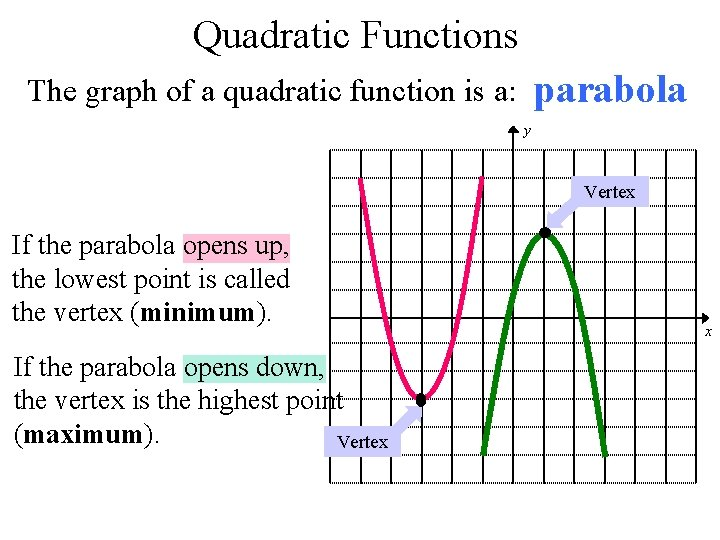Quadratic Functions parabola The graph of a quadratic function is a: y Vertex If the parabola opens up, the lowest point is called the vertex (minimum). If the parabola opens down, the vertex is the highest point (maximum). Vertex x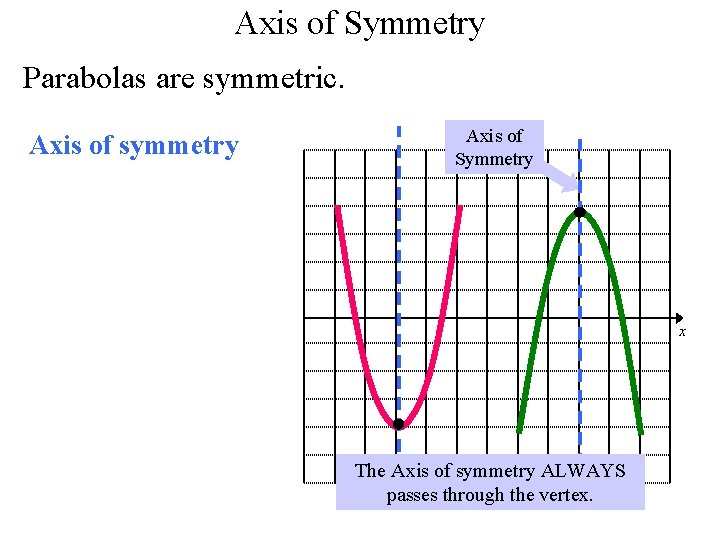Axis of Symmetry Parabolas are symmetric. Axis of symmetry Axisy of Symmetry x The Axis of symmetry ALWAYS passes through the vertex.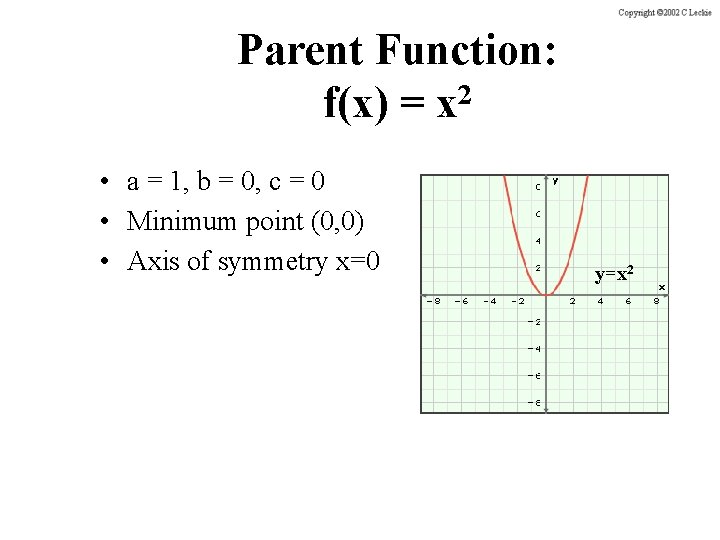Parent Function: 2 f(x) = x • a = 1, b = 0, c = 0 • Minimum point (0, 0) • Axis of symmetry x=0 y=x 2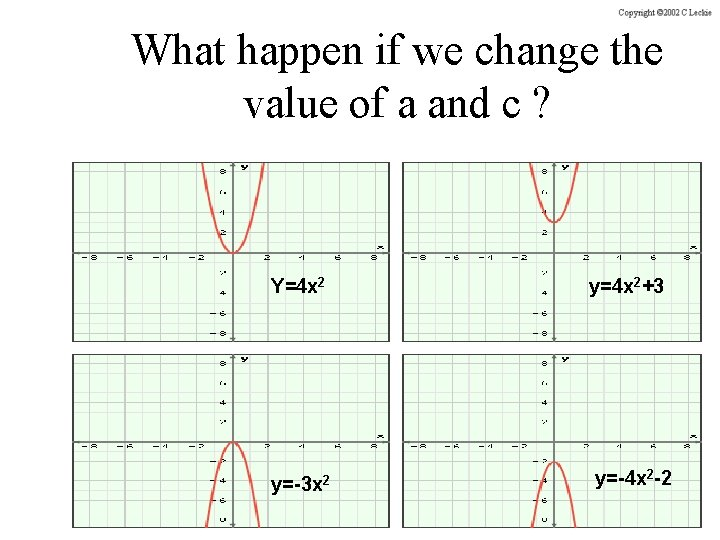What happen if we change the value of a and c ? Y=4 x 2 y=4 x 2+3 y=-3 x 2 y=-4 x 2 -2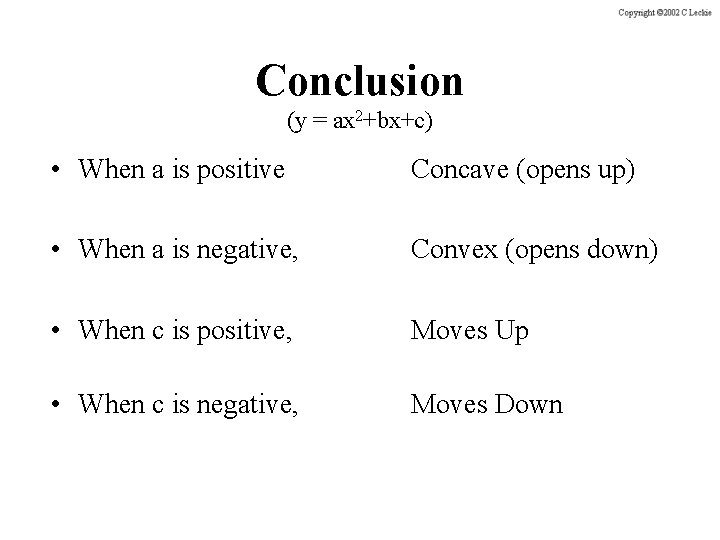Conclusion (y = ax 2+bx+c) • When a is positive Concave (opens up) • When a is negative, Convex (opens down) • When c is positive, Moves Up • When c is negative, Moves Downy = ax 2 + bx + c y a>0 x a<0Finding the Axis of Symmetry When a quadratic function is in standard form y = ax 2 + bx + c, the equation of the Axis of symmetry isSTEP 1: Find the Axis of symmetry y STEP 2: Make a table of 3 points Axis of Symmetry: Vertex: Min or Max? Roots: Y-intercept: x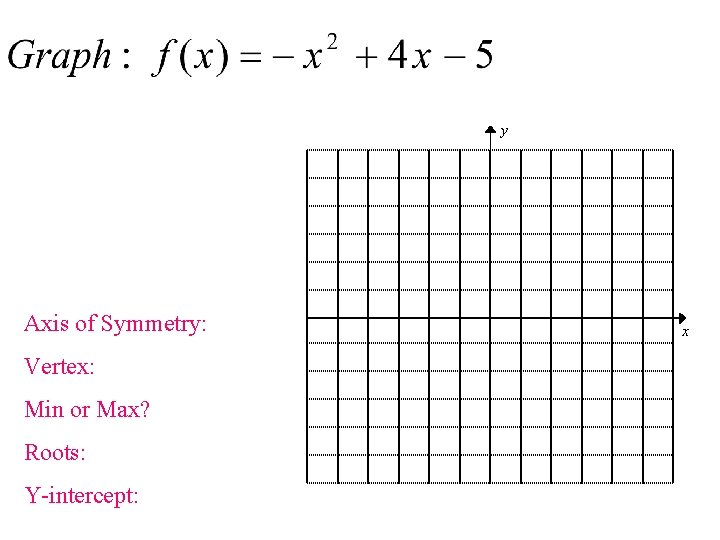y Axis of Symmetry: Vertex: Min or Max? Roots: Y-intercept: x